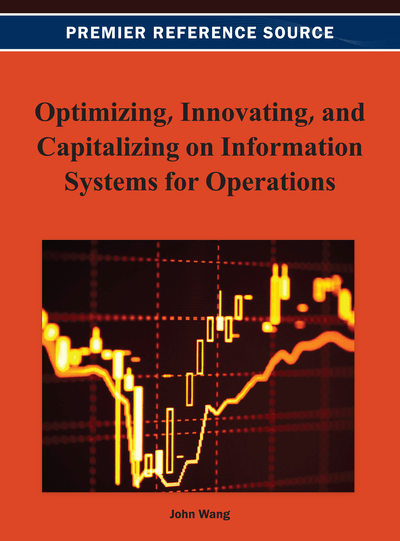# Local Perturbation Analysis of Linear Programming with Functional Relation Among Parameters

DOI: 10.4018/978-1-4666-2925-7.ch001
OnDemand:
(Individual Chapters)
Available
\$29.50
No Current Special Offers

## Abstract

In this paper, the authors study the sensitivity analysis for a class of linear programming (LP) problems with a functional relation among the objective function parameters or those of the right-hand side (RHS). The classical methods and standard sensitivity analysis software packages fail to function when a functional relation among the LP parameters prevail. In order to overcome this deficiency, the authors derive a series of sensitivity analysis formulae and devise corresponding algorithms for different groups of homogenous LP parameters. The validity of the derived formulae and devised algorithms is corroborated by open literature examples having linear as well as nonlinear functional relations between their vector b or vector c components.
Chapter Preview
Top

## Introduction

The main purpose of classical sensitivity analysis is to examine the variations of the objective function's optimal values and the solution components as a result of the infinitesimal changes in one of the parameters while the other ones are kept fixed (Bradley et al., 1977). One of the problems confronted in classical sensitivity analysis is when several parameters are changed simultaneously. In those cases, classical methods cannot obtain the effect of the perturbations on the objective function as well as on the optimal solution because when the simultaneous changes happen in the amplitudes range of basic variables or binding constraints, the order of basic solution changes. However, there is a conservative bound for the objective function coefficients or the right-hand side (RHS) parameters on their simultaneous changes. This bound is introduced under the 100 percent rule of changes when the perturbed coefficients of objective function are related to basic variables, or when the RHS parameters are related to binding constraints. The 100 percent rule states that if the sum of the proper fraction of the desired changes to the maximum possible change in that direction is less than or equal to one then the current basic optimal solution will remain unchanged (Bradley et al., 1977).

The general form of linear programming (LP) problem can be considered as follows:

(1) whereis anmatrix with full rank andis a column vector called the RHS parameters resembling the amount of resources, andis the coefficient vector of the objective function; they are collectively called the parameters of the LP problem. A simplex algorithm may be used to solve the LP problems. A simplex algorithm, in each step, chooses a set of the independent columns from matrixprovides the correspondent basic feasible solutions and checks for the optimality conditions. It is assumed that a basic optimal solution is available for this problem.

Let us introduce the following mathematical notations and definitions used throughout this paper and for the simplex table:

## Complete Chapter List

Search this Book:
Reset